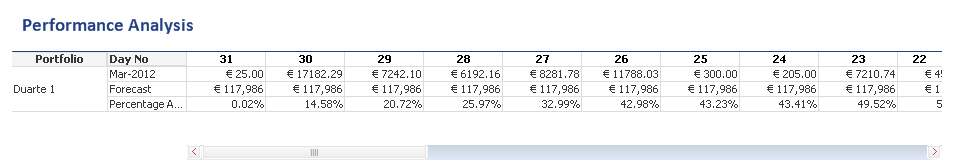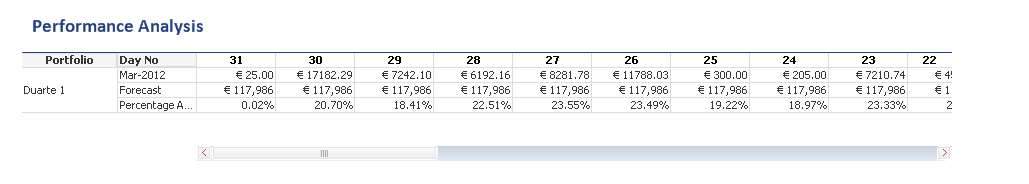# QlikView Layout & Visualizations

Discussion Board for collaboration on QlikView Layout & Visualizations.

HighlightedNot applicable

## Reversing the display of accumulated data in a table

I hope this is an interesting question, it is at the very least a bit of a challenge being thrown down.

I have a customer that wants a table that shows the sum of income by month, forecast figure and the percentage income against forecast (accumulated)Now I caan get it to work with the percentage accumulating left to right...The customer however wants to see this running in reverse, but without affecting the sort order of the rest of the table.

=rangesum(Before(Sum ( {< MonthSerial = {\$(=max(C_MonthSerial))}  >}  Collection_Week)/max ( {< MonthSerial = {\$(=max(C_MonthSerial))}  >}  Forecast_Amt),0, ColumnNo()))

I foolishly thought that I could just swith the Before to After and I would get the desired affect however I get the folllowing:Am I missing something? or is it a case of it not being possible? or stupidly complicated?

1 Solution

Accepted Solutions
HighlightedMVP & Luminary

## Re: Reversing the display of accumulated data in a table

Would that max percentage perhaps be...

Sum ( {< MonthSerial = {\$(=max(C_MonthSerial))}  >} total Collection_Week)/max ( {< MonthSerial = {\$(=max(C_MonthSerial))}  >} total Forecast_Amt)

...?

talk is cheap, supply exceeds demand
4 Replies
HighlightedMVP & Luminary

## Re: Reversing the display of accumulated data in a table

How about subtracting it from 1:

=1-rangesum(Before(Sum ( {< MonthSerial = {\$(=max(C_MonthSerial))}  >}  Collection_Week)/max ( {< MonthSerial = {\$(=max(C_MonthSerial))}  >}  Forecast_Amt),0, ColumnNo()))

talk is cheap, supply exceeds demand
HighlightedNot applicable

## Re: Reversing the display of accumulated data in a table

 Gysbert Wassenaar wrote:How about subtracting it from 1:=1-rangesum(Before(Sum ( {< MonthSerial = {\$(=max(C_MonthSerial))}  >}  Collection_Week)/max ( {< MonthSerial = {\$(=max(C_MonthSerial))}  >}  Forecast_Amt),0, ColumnNo()))

Ok, that is really helpful, my only issue is that the percentage can be more than 100%...So I need to somehow substitute the 1 for the maximum percentage in the dimensions?.

HighlightedMVP & Luminary

## Re: Reversing the display of accumulated data in a table

Would that max percentage perhaps be...

Sum ( {< MonthSerial = {\$(=max(C_MonthSerial))}  >} total Collection_Week)/max ( {< MonthSerial = {\$(=max(C_MonthSerial))}  >} total Forecast_Amt)

...?

talk is cheap, supply exceeds demand
HighlightedNot applicable

## Re: Reversing the display of accumulated data in a table

 Gysbert Wassenaar wrote:Would that max percentage perhaps be...Sum ( {< MonthSerial = {\$(=max(C_MonthSerial))}  >} total Collection_Week)/max ( {< MonthSerial = {\$(=max(C_MonthSerial))}  >} total Forecast_Amt)...?

I'm going for =Sum ( {< MonthSerial = {\$(=max(C_MonthSerial))}  >} Total Collection_Week)/max ( {< MonthSerial = {\$(=max(C_MonthSerial))}  >}  Forecast_Amt)-rangesum(Before(Sum ( {< MonthSerial = {\$(=max(C_MonthSerial))}  >}  Collection_Week)/max ( {< MonthSerial = {\$(=max(C_MonthSerial))}  >}  Forecast_Amt),0, ColumnNo()))